@lutingting 2016-11-04T08:15:11.000000Z 字数 10899 阅读 8556

# SIFT特征提取及匹配

数字图像处理 图像特征提取

# 1.SIFT（Scale-invariant feature transform）算子的核心思想

• 利用不同尺度的高斯核函数对图像进行平滑，即构造图像的尺度空间
• 比较不同尺度平滑后的图像差别，在某局部范围内，差别最大或者差别最小的像素点就是特征明显的点

• 尺度不变性：在尺度空间内进行的特征点检测

# 2.什么是尺度空间呢？

## 2.1 一篇百度文库的文章关于尺度空间的分析

### 例子1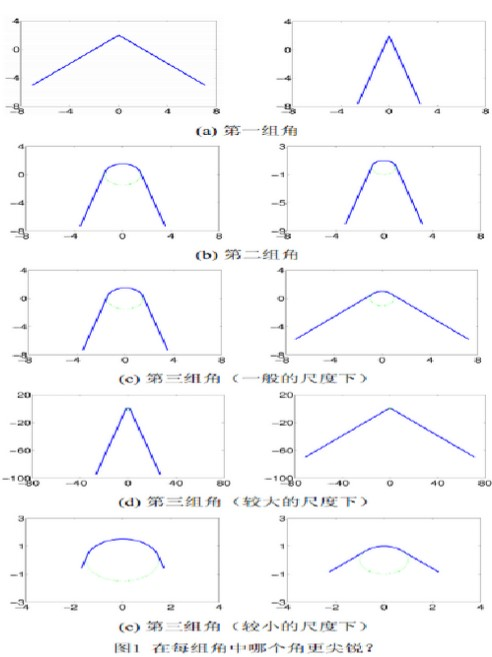• 图1（a）中，显然右边的角更尖锐，这是因为同左边的角相比其角度值较小
• 图1（b）中，也是右边的角更尖锐，这是因为同左边的角相比其曲率值较大
• 图1（c）中，问题的答案则要困难得多。左边的角一方面具有较小的角度值（因此更尖锐），另一方面又具有较小的曲率值（因此更圆钝）。右边的角情形刚好相反，一方面因为具有较大的角度值更圆钝，另一方面又因为具有较大的曲率值显得更尖锐。事实上，本问题对于图1（a）和图1（b）来说是纯粹的数学问题，依据数学上的基本概念（即角度、曲率）便可以做出判断。而图1（c）中两个角之间的比较已经不再是纯粹的数学问题，在数学上没有明确的答案。确切地说，这是一个尺度空间中的视觉问题，其答案取决于问题所在的“尺度”而不是某个数学指标。这里，“尺度”可以被直观地理解为观察窗口的大小。在图1（c）中，我们观察两个角的窗口大小都是$12*16$
• 图1（d）中，调整了观察窗口，其大小变成$120*160$（假设所比较的两个角都具有无限长的边）。在这个较大的尺度下，问题的答案变得非常明朗：左边的角更加尖锐。
• 图1（e）中，观察窗口的大小变更为$6*8$在这个较小的尺度下，问题的答案发生了有趣的变化：此时右边的角更加尖锐。

### 例子2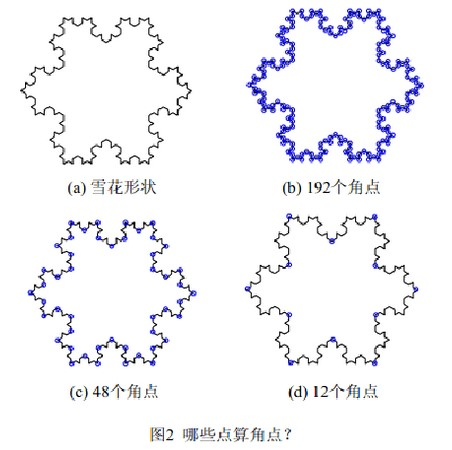• 图2(a) 呈现了一片雪花的形状轮廓，要求我们找出该形状上的角点。在很多计算机视觉任务中，角点都有着重要的作用。数学上，角点一般是指大曲率点或曲率无穷大点。
• 在图2(b)中，雪花形状上所有曲率无穷大点都被确认为角点，一共有192个，如圆圈所标记。这个答案在数学上无疑是正确的、完美的令人惊奇的，但它对于完成一个视觉任务（比如理解和分析这个形状）来说并没有多大的意义。
• 如果我们仅选择图2(c)中所标记出的48个点作为角点，感觉上要更好点。作为图2(b)中所标记的192个角点中的一部分，这48个角点在理解和分析雪花形状的结构时要比其余的角点具有更高的重要性。
• 实际上，按照这一思路，我们不难发现在这48个角点中又有12个角点其重要性还要更高一些，如图2(d)中所标记。

## 2.2 SIFT中的尺度空间的概念

• David Lowe关于Sfit算法，2004年发表在Int. Journal of Computer Vision的经典论文中，对尺度空间（scale space)是这样定义的

It has been shown by Koenderink (1984) and Lindeberg (1994) that under a variety of reasonable assumptions the only possible scale-space kernel is the Gaussian function. Therefore,the scale space of an image is defined as a function, L(x; y; sigma) that is produced from the convolution of a variable-scale Gaussian, G(x; y; sigma), with an input image, I(x; y)

• 从上面的定义可以看到：

• 高斯卷积核是实现尺度变换的唯一变换核
• 一幅图像的尺度空间被定义为：高斯卷积核与该图像的卷积，它是高斯卷积核中的参数$\sigma$的函数，这里用到“空间”这个词，是因为$\sigma$是可以连续变化的，具体地，图像$I(x,y)$的尺度空间为：

其中：

• $G(x,y,\sigma) = \frac{1}{2\pi\sigma^2}*e^{-\frac{x^2+y^2}{2\sigma^2}}$是高斯核函数
• $\sigma$是尺度因子，$\sigma$的大小决定图像的平滑程度，大尺度对应图像的概貌特征，小尺度对应图像的细节特征。大的$\sigma$值对应粗糙尺度(低分辨率)，小的$\sigma$值对应精细尺度(高分辨率)。
• $*$是卷积操作
• $L(x,y,\sigma)$就是输入图像$I(x,y)$的尺度空间。

# 3.SIFT特征提取

• 尺度空间极值检测
• 关键点位置及尺度确定
• 关键点方向确定
• 特征向量生成

## 3.1 尺度空间极值检测

SIFT特征点其实就是尺度空间中稳定的点/极值点，那么，为了得到这些稳定点：

• 首先，需要对输入图像建立尺度空间（通过图像高斯金字塔）
• 然后，需要从建立得到的尺度空间中检测极值点（转换为图像差分高斯金字塔中极值点的检测）
• 获取差分高斯金字塔
• DoG中极值点的检测

### 3.1.1 尺度空间的建立（高斯金字塔的建立）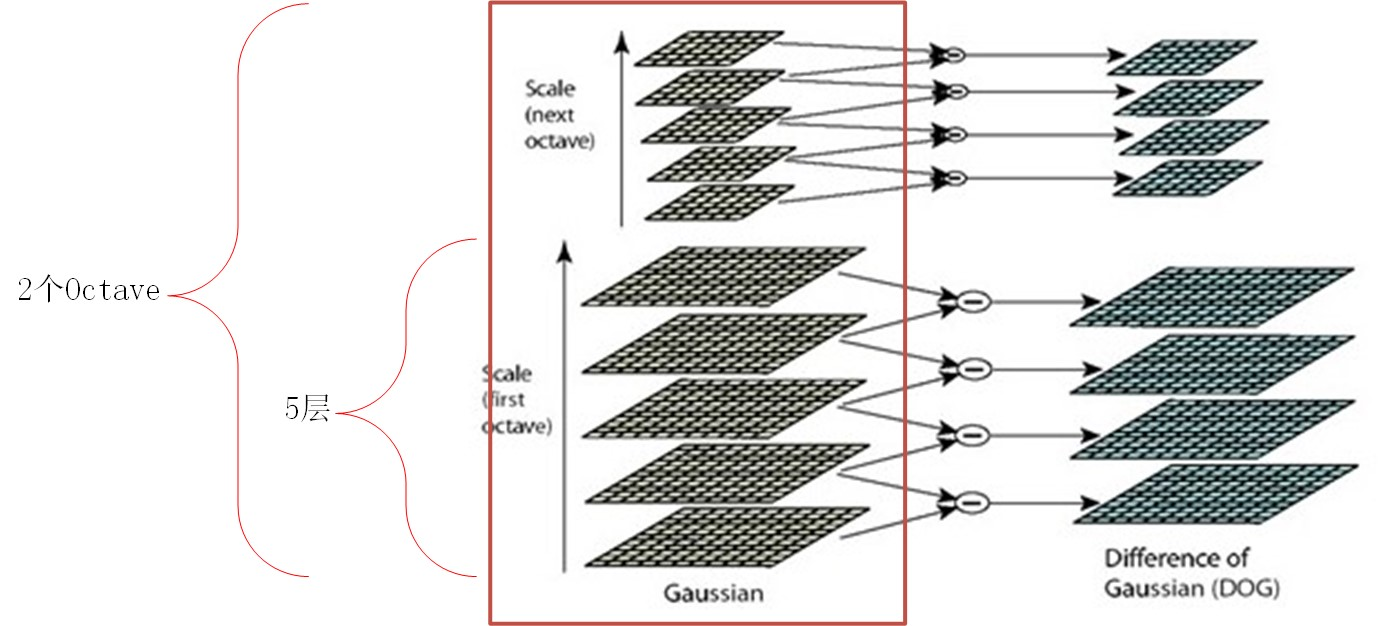• 高斯金字塔分为S组（即S个塔、或者称为S个octave），每个塔中有s层；具体有几个塔、每个塔包含几层由输入图像的尺寸决定！对于上图而言：有S=2组，每组由s=5层；
• 同一塔中图像尺寸相同但尺度不同、不同塔中图像尺寸和尺度都不同（注意：这里的尺寸和尺度概念不同！尺寸对应图像分辨率、尺度为高斯核大小）

• 每一个塔中图像尺寸确定方法为：第一个塔的尺寸为原图大小，后面每个塔的尺寸为上一个塔的降采样的结果（即长宽分别减半的结果）
• 每个塔中各层图像的尺度不同，即$\sigma$取值不同，各层之间相差一个$k$值。例如：上图中第一个塔中各层的尺度因子分别为：$\sigma$$k\sigma$$k^2\sigma$$k^3\sigma$$k^4\sigma$；而第二个塔中各层的尺度因子分别为：$2\sigma$$2k\sigma$$2k^2\sigma$$2k^3\sigma$$2k^4\sigma$
• 如何确定相邻层之间的比例因子k呢？下图是同一Octave中不同层和不同Octave之间的尺度大小关系，为了满足尺度变化的连续性，即某一组的最后一层对应的尺度与下一组的第一层对应的尺度应该相差一个k倍，所以应该有$k^{s-1}\sigma_0 *k = 2*\sigma_0$，所以，$k^s=2$，即$k=2^{1/2}$，其中，$\sigma_0$为基础尺度因子。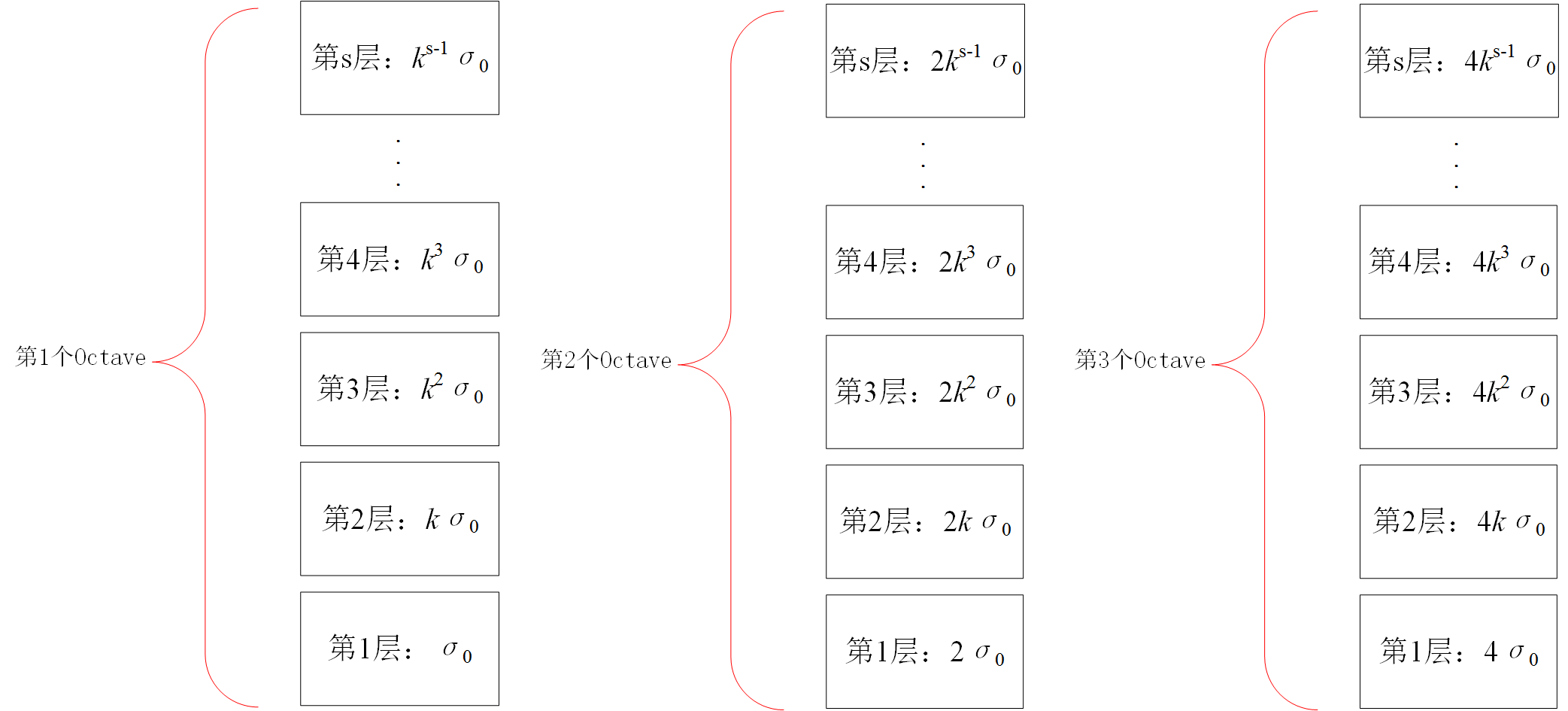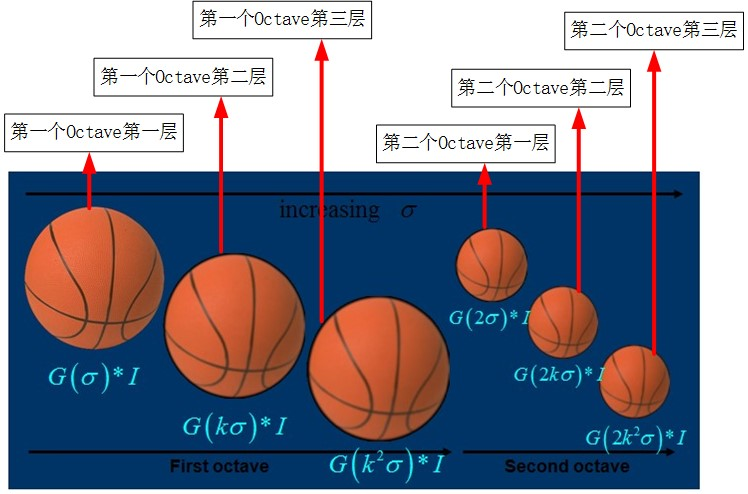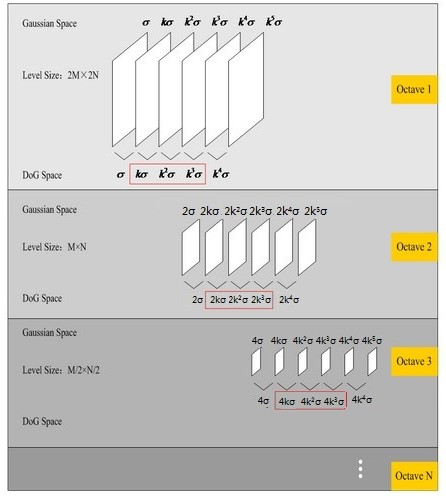%读入图像并将其转换成灰度图像 I=imread('qingdao.jpg');I=rgb2gray(I);% 利用尺度为3*3的高斯核进行滤波w1=fspecial('gaussian',3,0.5);g1=imfilter(I,w1,'conv','symmetric','same');% 利用尺度为11*11的高斯核进行滤波w2=fspecial('gaussian',11,0.5);g2=imfilter(I,w2,'conv','symmetric','same');% 显示处理结果figure;subplot(1,3,1);imshow(I);title('原始图像');subplot(1,3,2);imshow(g1);title('3*3高斯');subplot(1,3,3);imshow(g2);title('11*1高斯');
• matlab中利用fspecial函数产生离散高斯核：H=fspecial('gaussian',hsize,sigma)
• H是一个矩阵，它是由离散高斯卷积核计算得到的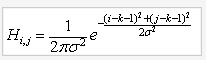• 它是利用二维高斯函数$G(x,y,\sigma) = \frac{1}{2\pi\sigma^2}*e^{-\frac{x^2+y^2}{2\sigma^2}}$计算得到的
• 其中的hsize为高斯核的大小，sigma为高斯核的标准偏差，即高斯核的大小

### 3.1.2 图像差分高斯金字塔（DoG）的建立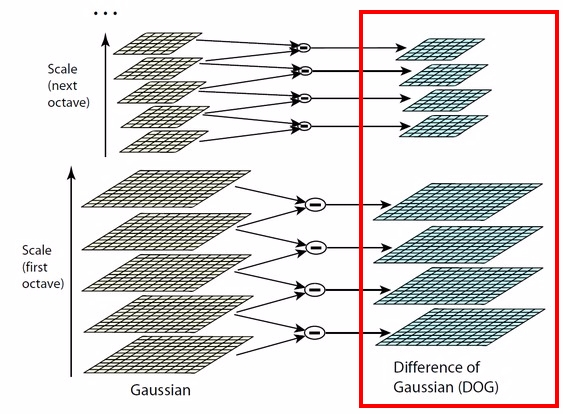### 3.1.3 尺度空间中特征点的检测（DoG中极值点的检测）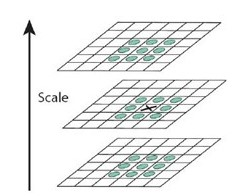• 那么按照上图可得Gauss Space和DoG space 分别有3个（s个）和2个（s-1个）分量，在DoG space中，1st-octave两项分别是σ,kσ; 2nd-octave两项分别是2σ,2kσ;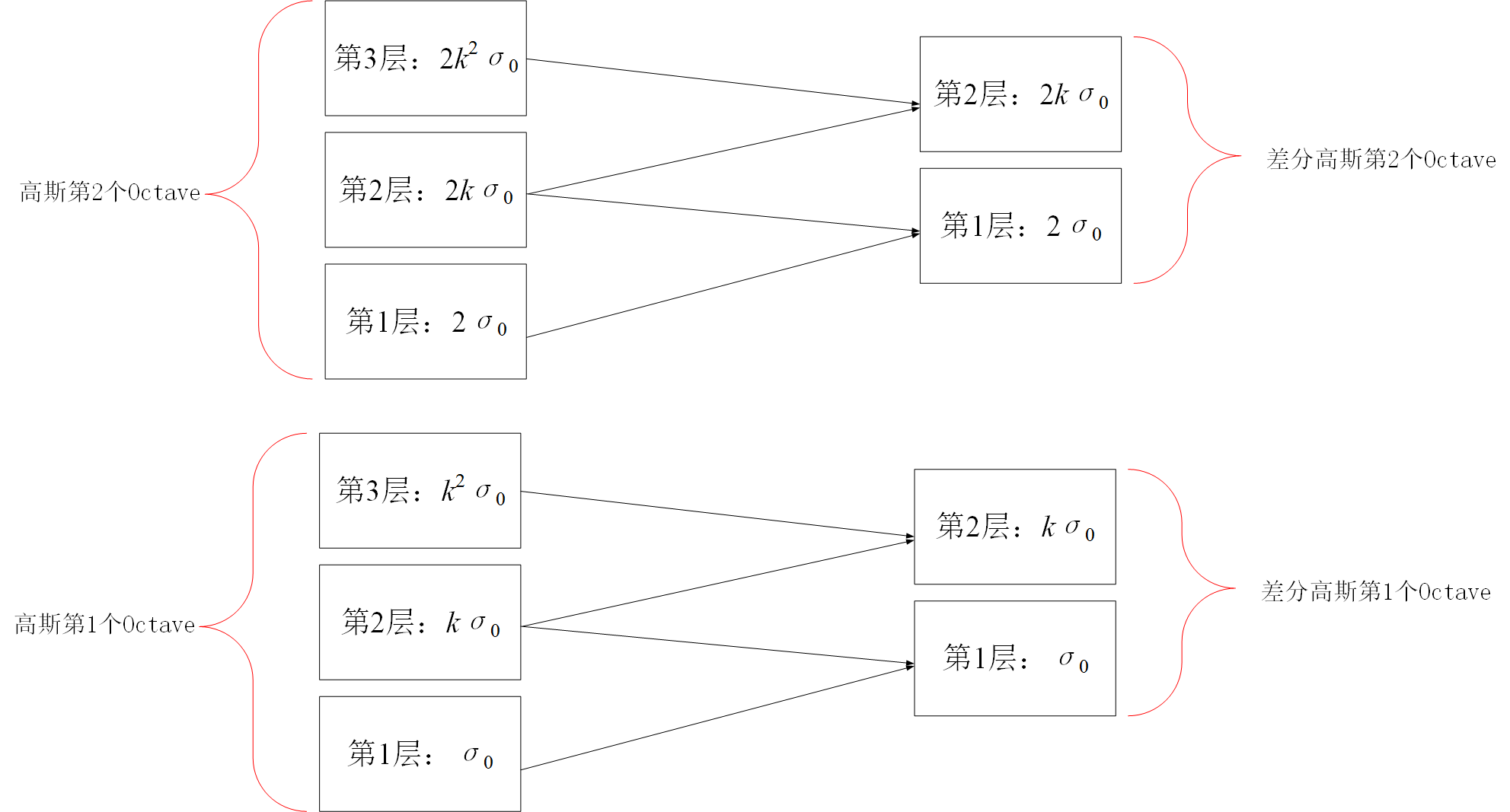• 由于无法比较极值，我们必须在高斯空间继续添加高斯模糊项，使得DoG中第一个Octave形成σ,kσ,k2σ,k3σ,k4σ，这样就可以选择中间三项kσ,k2σ,k3σ（只有左右都有才能有极值）；那么下一octave中（由上一层降采样获得）所得三项即为2kσ,2k2σ,2k3σ，其首项2kσ=24/3。刚好与上一octave末项k3σ=23/3尺度变化连续起来，所以每次要在Gaussian space添加3项，每组（塔）共S+3层图像，相应的DoG金字塔有S+2层图像。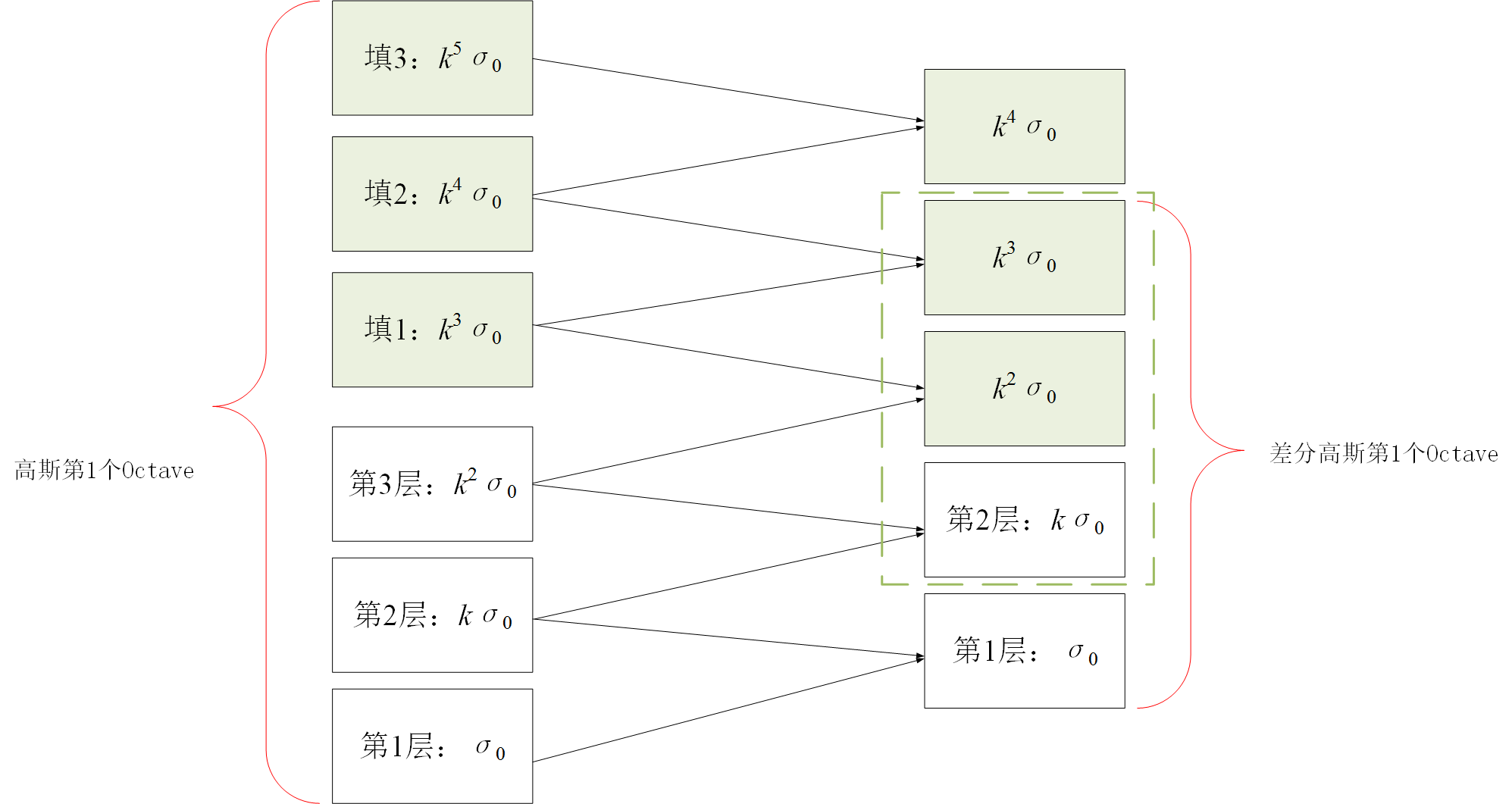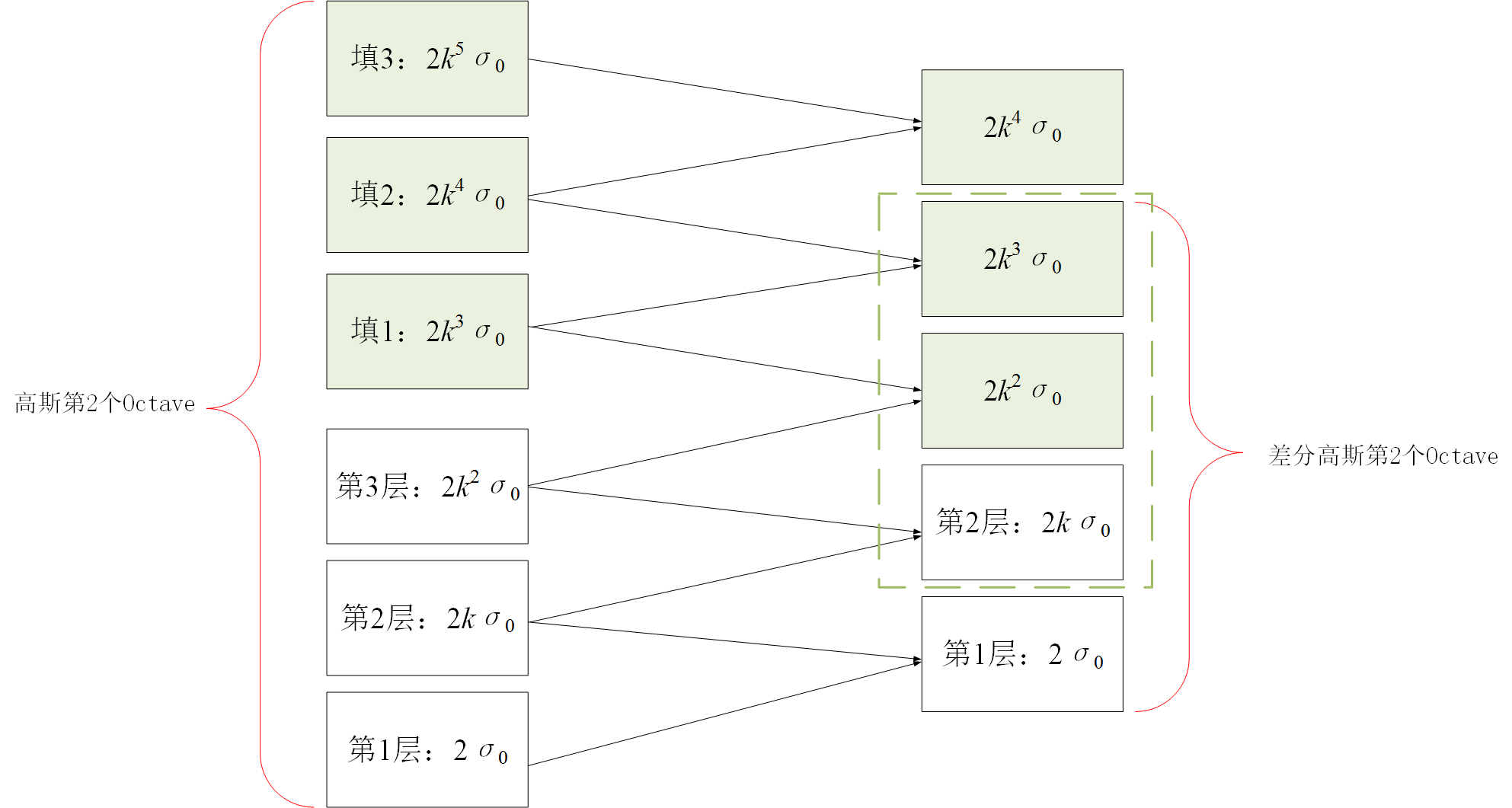• 剔除对比度低的点
• 剔除边缘点

## 3.3 关键点方向确定

• 计算关键点的方向，需要利用以该关键点为中心的某邻域窗口内所有像素点的梯度分布特性（目的是为了使的sift算子具备旋转不变性），所以，下面首先给出计算尺度空间中每个像素点的梯度模值和方向的方法，按照下面公式计算：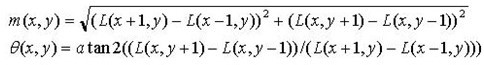• 其中L所用的尺度为每个关键点各自所在的尺度。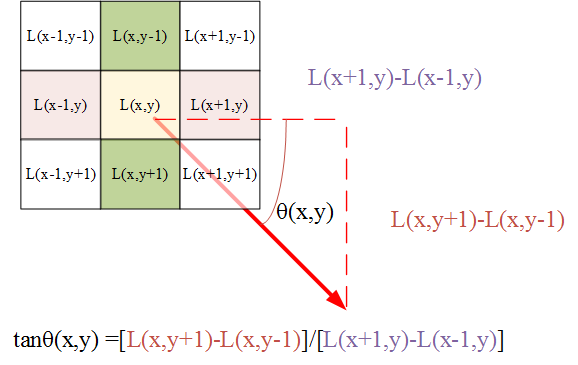• 接下来，对于每个关键点（假设尺度为sigma），利用直方图统计其相邻窗口内的像素的梯度分布，从而，确定该关键点的方向，具体方法如下：

• 分别计算以该关键点为中心的相邻窗口中像素点的梯度方向和模值
• 为该窗口内每一个像素点赋予一个权值：由每个像素点对应的梯度模值和以关键点为中心尺度为1.5sigma的高斯核决定
• 设定某种规则，开始统计直方图，例如，这里将方向（0~360°）分为8份，那么，梯度方向直方图将有8个柱，窗口内每个像素到底属于哪个柱由该像素点的梯度方向确定（见下图右侧子图所示）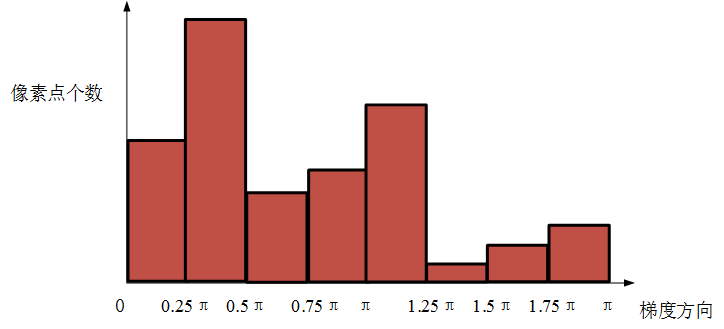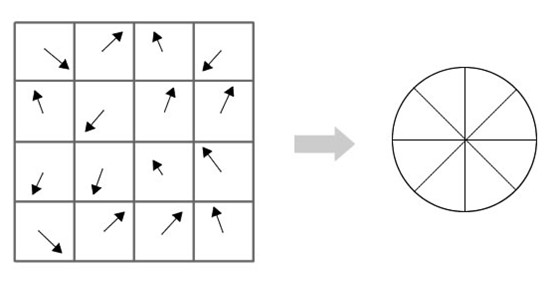• 在该关键点对应的梯度方向直方图中，找到包含像素点最多的那个方向作为该关键点的方向，对于上图而言，方向角(0.25π,0.5π)这个区间内的像素点最多，所以，以(0.25π+0.5π)/2 = 0.375π作为该关键点的方向

## 3.4 特征向量生成

• 将坐标轴旋转到关键点的方向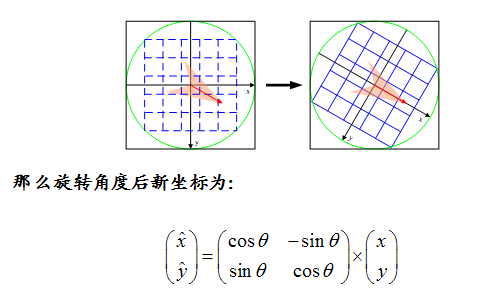• 对于某一个关键点，取其周围$8*8$窗口，如下图绿色区域所示，其中，$8*8$窗口内每个小矩形框为一个像素，箭头表示该像素位置的梯度方向和模值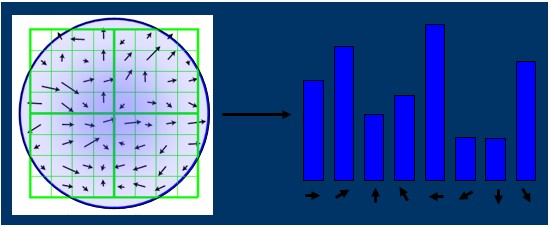• 在该$8*8$窗口对应的4个（称为4个种子点）$4*4$的小块儿上，分别计算该小块儿包含的16个像素点的梯度直方图（8个柱），并进行累加（每个柱对应的所有像素点的梯度模值累加），每个小块儿可以得到8个特征（8个方向对应的模值的累加），从而，4个种子点将得到关键点的4*8=32个特征，如下图右侧所示，4个种子点，每个种子点产生8个特征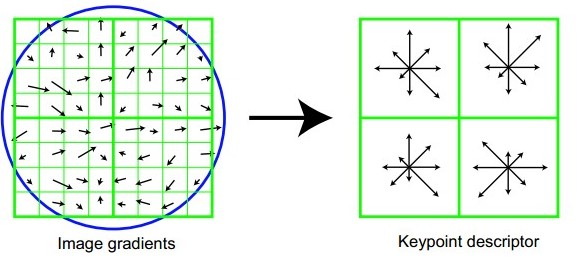• 实际中，Lowe建议使用$4*4$个子块儿（称为16个种子点）进行特征计算，那么，每个关键点将具有16*8=128个特征，如下图所示，此时，需要在特征点周围取$16*16$的窗口，分割为$4*4$个子块儿（这样做的目的是为了增强后续匹配的稳健性）。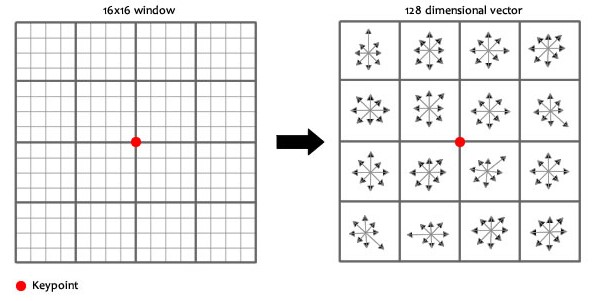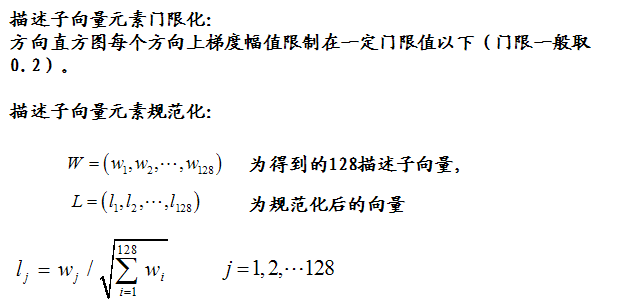# 4.SIFT特征的匹配

• 取图像A中的某个关键点，并找出其与图像B中欧式距离最近的前两个关键点
• 在这两个关键点中，如果最近的距离除以次近的距离少于某个比例阈值，则接受这一对匹配点。降低这个比例阈值，SIFT匹配点数目会减少，但更加稳定。
• 利用2个近邻点比较的目的是为了排除因为图像遮挡和背景混乱而产生的无匹配关系的关键点，所以Lowe提出了比较最近邻距离与次近邻距离的方法,距离比率ratio小于某个阈值的认为是正确匹配。因为对于错误匹配,由于特征空间的高维性,相似的距离可能有大量其他的错误匹配,从而它的ratio值比较高。Lowe推荐ratio的阈值为0.8。但作者对大量任意存在尺度、旋转和亮度变化的两幅图片进行匹配，结果表明ratio取值在0. 4~0. 6之间最佳，小于0. 4的很少有匹配点，大于0. 6的则存在大量错误匹配点。(如果这个地方你要改进，最好给出一个匹配率和ration之间的关系图，这样才有说服力)，作者建议ratio的取值原则如下:
• ratio=0. 4　对于准确度要求高的匹配；
• ratio=0. 6　对于匹配点数目要求比较多的匹配；
• ratio=0. 5　一般情况下。
• 也可按如下原则: 当最近邻距离<200时，ratio=0. 6；反之，ratio=0. 4。ratio的取值策略能排分错误匹配点。

Reference
1. SIFT keypoint detector实现代码：http://www.cs.ubc.ca/~lowe/keypoints/
2. David Lowe个人主页：http://www.cs.ubc.ca/~lowe/home.html
3. David Lowe关于SIFT的论文2004《Distinctive Image Features
from Scale-Invariant Keypoints》pdf版本：http://www.cs.ubc.ca/~lowe/papers/ijcv04.pdf
5. Rachel-Zhang博客-SIFT特征提取分析. http://blog.csdn.net/abcjennifer/article/details/7639681
6. http://www.zhihu.com/question/19911080
7. http://blog.csdn.net/songzitea/article/details/16986423

# 5.下面是一些参考程序

## 5.1

%size为模板大小%sigma为标准差size = 3;sigma =3 ;%下面的代码其实是从fspecial中摘录出来的，我做了一些更改放到自己写的函数里面便于解释%计算高斯模板的中心位置siz    = ([size size]-1)/2;sig    = sigma;%用meshgrid是为了加速，不用for循环[x y]  = meshgrid(-siz(2):siz(2),-siz(1):siz(1));%计算exp(-(x^2+y^2)/(2*sig^2))%我想你肯定有一个疑问，那就是为什么不除以2*pi*sig^2%因为不除也没有关系，因为最后归一化之后会约掉arg    = -(x.*x+y.*y)./(2*sig*sig);h      = exp(arg);%不知道它为什么要这样，忘懂得人解释一下h(h<eps*max(h(:))) = 0;%求和，用来归一化sumh   = sum(h(:));%防止求和之后出现为0的情况，然后再归一化一下使高斯，模板为小数if sumh ~=0    h=h/sumh;end%% 绘制二维高斯曲面u=[-10:0.1:10];v=[-10:0.1:10];[U,V]=meshgrid(u,v);H=1/2/pi/sigma*exp(-(U.^2+V.^2)./2/sigma^2);mesh(u,v,H); %绘制三维曲面的函数title('高斯函数曲面');%% 利用fspecial产生高斯核hold on;for i=1:3    for j=1:3             plot3(i-2,j-2,h(j,i),'r*');    endend

## 5.2

clear;clc;close all%% 输入图像，并归一化到统一的尺寸row=256;colum=256;img=imread('lenna.png');img=imresize(img,[row,colum]);img=rgb2gray(img);img=im2double(img);origin=img;%% 下面分别利用不同的sigma值对输入图像进行卷积操作sigma0 = sqrt(2);level = 3;for i=1:level    scale=sigma0*sqrt(2)^(1/level)^((i-1)*level+i);    f=fspecial('gaussian',[1,floor(6*scale)],scale);    image_output=conv2(origin,f','same');    figure;imshow(image_output);hold on;    title(['scale = ',num2str(scale),',and size of kernel is ',num2str(size(f,1)),'*',num2str(size(f,2))]);end%% 绘制二维高斯曲面sigma = 3;u=[-10:0.1:10];v=[-10:0.1:10];[U,V]=meshgrid(u,v);H=1/2/pi/sigma*exp(-(U.^2+V.^2)./2/sigma^2);mesh(u,v,H); %绘制三维曲面的函数title('高斯函数曲面');%% 利用fspecial产生高斯核f=fspecial('gaussian',[3,3],sigma);hold on;for i=1:3    for j=1:3             plot3(i,j,f(j,i),'r*');    endend• 私有
• 公开
• 删除